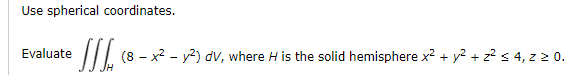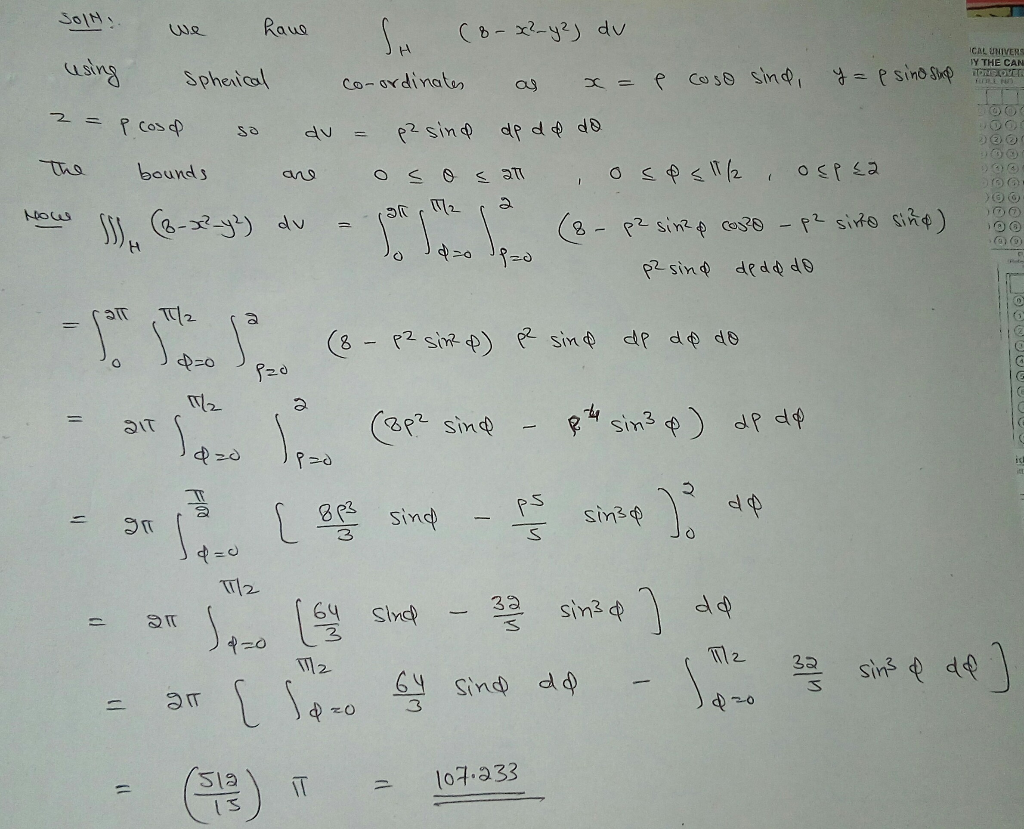Homework Help Question & Answers

# Use spherical coordinates. Evaluate (8 -x2 -v-) dvV, where H is the solid hemispherexy2 4, 0. J JHUse spherical coordinates. Evaluate (8 -x2 -v-) dvV, where H is the solid hemispherexy2 4, 0. J JH

#### Homework Answers

ReportAnswer #1Add Homework Help Answer
##### Add Answer of: Use spherical coordinates. Evaluate (8 -x2 -v-) dvV, where H is the solid hemispherexy2 4, 0. J JH
Your Answer: Your Name: What's your source?
Not the answer you're looking for? Ask your own homework help question. Our experts will answer your question WITHIN MINUTES for Free.
More Homework Help Questions Additional questions in this topic.

• #### use spherical coordinates to evaluate the triple integral of (e^-(x^2+y^2+z^2))/(sqrt(x^2+y^2+z^2)dV where E is the region bounded by the spheres x^2+y^2+z^2=49 and x^2+y^2+z^2=81

Need Online Homework Help?

Get FREE EXPERT Answers
WITHIN MINUTES
Related Questions